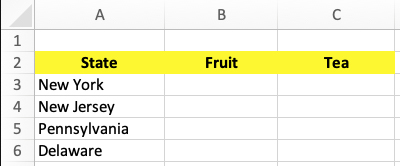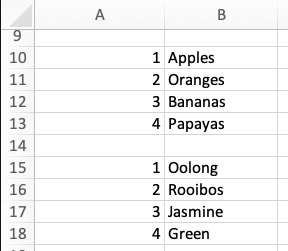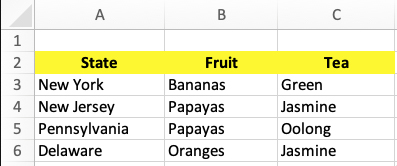# How to randomize columns of imported data in Excel

Published on
3,302 Points
202 Views
1 Endorsement
Using a Vlookup with one of Excel's random number functions to create randomized columns of data from pre-defined lists.

Someone recently asked me how to create a table that has columns of data from a fixed set of entries, but the entries should be randomized. There are undoubtedly several ways of doing this, but I decided that combining a Lookup table with a random number generator would be the easiest way. It also makes it easy to add items to the data source.

Here is the table before we import the data. We have two columns for data, and the contents of each column will get filled from elsewhere. Each time the sheet recalculates, the random number generator runs and the results will be different.

But first, let’s set up the table so it looks like this:Now create your data sources, one source for each of the two columns. If you have a lot of data, put each one on a separate sheet. For simplicity, we will keep the two sources on the same sheet as the main table. Each column of data gets a corresponding column containing unique index numbers, and this numbered column must be on the left:The Vlookup function will read the data from each source into the main table. The syntax of the function is:

`=VLOOKUP(lookup value, table array, column number, exact/approximate match)`

Since the data source of the first column has 4 numbered entries, the lookup value will be a random number from 1 through 4. To do that, let’s nest the Randbetween function inside the Vlookup function. The syntax is:

`=RANDBETWEEN(starting integer, ending integer)`

To fill the Fruit column, the Vlookup’s table array is A10:B13. It must be an absolute reference, so we’ll write it as \$A\$10:\$B\$13. If you aren’t familiar with absolute references, watch my tutorial on YouTube. The column number is 2 because that’s the column where the varieties of fruit and tea are, and we want an exact match because that’s the type of data we have.

So in the first blank cell of the main table (B3 in this example), enter this formula:

`=VLOOKUP(RANDBETWEEN(1,4),\$A\$10:\$B\$13,2,FALSE)`

AutoFill down to row 6.

Now use the same technique for the tea column. Start with this formula in C3:

`=VLOOKUP(RANDBETWEEN(1,4),\$A\$15:\$B\$18,2,FALSE)`

AutoFill down to row 6.You can test the formulas by doing something that makes the sheet recalculate, like write or modify a formula or edit data. You can also force the sheet to recalculate by pressing the F9 key in Windows or Fn + F9 on the Mac. Each time you do, the contents of the Fruit and Tea columns will change.